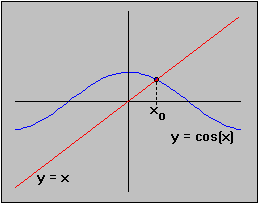#Interactive Real Analysis

Next | Previous | Glossary | Map

## 6.4. Topology and Continuity

### Examples 6.4.9(a):

Show that the equation cos(x) = x has a solution in the interval [-10, 10].
Let's take a look at the two function f(x) = cos(x) and g(x) = x in one coordinate system:So one can clearly see that there is exactly one solution. We can use Bolzano's theorem to actually prove that there must be at least one solution: Let h(x) = cos(x) - x. Then h is a continuous function and
h(-) = -1 +> 0
h() = -1 -< 0
Hence, by Bolzano's theorem there must be at least one place x0 where h( x0) = 0, or equivalently where cos( x0) = x0.

One can use Bolzano's theorem to construct an algorithm that will find zeros of a function to a prescribed degree of accuracy in many cases. In simple terms:

• start with an interval [a, b] where h(a) * h(b) < 0 (i.e. h(a) and h(b) have opposite signs)
• find a point c - usually (a + b) / 2 - such that either h(a) * h(c) < 0 or h(b) * h(c) < 0
• if h(a) * h(c) < 0, repeat this procedure with b replaced by c
• if h(b) * h(c) < 0, repeat this procedure with a replaced by c.
• Continue until the difference b - a is small enough.

Would this procedure find the zero of the function f(x) = x2 in the interval [-1, 1] ?

Next | Previous | Glossary | Map